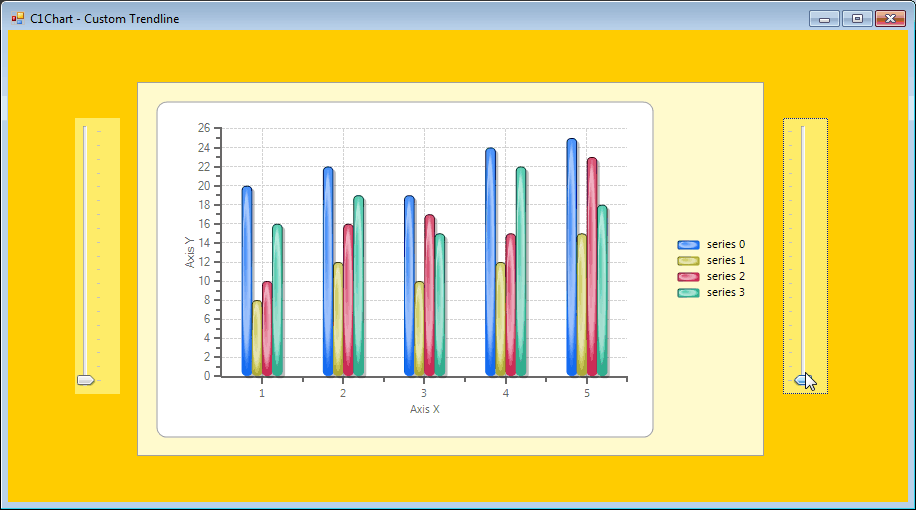﻿ Dynamic Trendlines in C1Chart# Dynamic Trendlines in C1Chart

Trendlines indicate the general course or tendency of something or a set of points on a Chart. The feature is extensively useful while displaying or analysing the data on charts. Using ComponentOne Chart for Winform you can create two types of Trendlines : 1. Static 2. Dynamic

## I) How To : Create Static Trendlines in C1Chart

The documentation link describes the same in detail. Here you need to pass a set of predefined values to the Trendline object before adding it to the C1Chart's TrendsList collection.

## II) How To : Create Dynamic Trendlines in C1Chart

Here, we will describe how one can create Dynamic Trendlines in C1Chart by dynamically taking the values from the Trackbars placed on the form. Hence, in this blog, we will be implementing a scenario where we will synchronize the Trackbars with the Custom Trendlines on C1Chart object.## Creating Custom Trendlines

To implement a custom trendline, a class implementing ICustomTrendLine interface is created whose instance is then assigned to CustomTrendLine property of a TrendLine object added to the C1Chart's TrendsList collection.

``````public class CustomTrendLine : C1.Win.C1Chart.ICustomTrendLine
{
private double[] _x;

private double[] _y;

public void Calculate(C1.Win.C1Chart.TrendLine tl, double[] x, double[] y)
{

if (x == null || x.Length == 0 || y == null || y.Length == 0)
{
\_x = null; \_y = null;
return;
}

// find min and max
double xmin = x, xmax = x;
double ymin1 = y, ymax1 = y;

ChartTrendline ff = new ChartTrendline();
xmin = ff.c1Chart1.ChartArea.AxisX.Min;
xmax = ff.c1Chart1.ChartArea.AxisX.Max;

ymin1 = ChartTrendline.y1();
ymax1 = ChartTrendline.y2();

_x = new double;
_y = new double;

\_x = xmin; \_y = ymin1;
\_x = xmax; \_y = ymax1;
}

public double[] GetXValues() { return _x; }

public double[] GetYValues() { return _y; }

// don't use it just return something

public double GetY(double x) { return 0; }

public string Text { get { return &quot;Custom trend&quot;; } }

}
``````

## Tracking the Values on TrackBars

Here we need to handle the ValueChanged and MouseUp events of the TrackBars to keep track of the current values. These values will be then used to plot the Custom Trendline on the chart.

``````//Create static variables used to plot Custom
//Trendlines to track the current on the TrackBars
static bool bar1 = false;
static int a = 0,b = 0;
public static int y1()
{
if (bar1 == true)
return b;
else
return a;
}

public static int y2()
{
if (bar1 == true)
return a;
else
return b;
}
bool draw = false;
//Capture the Trackbar Values
private void trackBar1_ValueChanged(object sender, EventArgs e)
{
bar1 = true;
draw = true;
a = trackBar1.Value;
b = trackBar2.Value;
}

private void trackBar1_MouseUp(object sender, MouseEventArgs e)
{
if (draw)
{
DrawLevelLine();
draw = false;
}
}

private void trackBar2_ValueChanged(object sender, EventArgs e)
{
bar1 = false;
draw = true;
a = trackBar1.Value;
b = trackBar2.Value;
}

private void trackBar2_MouseUp(object sender, MouseEventArgs e)
{
if (draw)
{
DrawLevelLine();
draw = false;
}
}
private void DrawLevelLine()
{
c1Chart1.ChartGroups.ChartData.TrendsList.Clear();
// create trend line
// setup line properties
tl.LineStyle.Color = Color.DarkRed;
tl.LineStyle.Thickness = 3;
tl.LineStyle.Pattern = C1.Win.C1Chart.LinePatternEnum.Dash;
// set custom trend line
tl.CustomTrendLine = new CustomTrendLine();
if(bar1 == true)
bar1 = false;
}
``````GrapeCity

GrapeCity Developer Tools